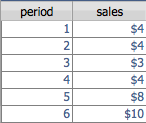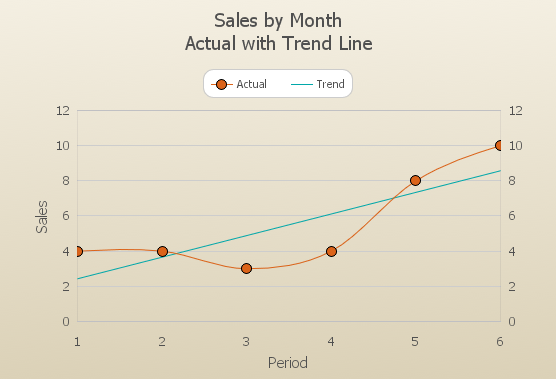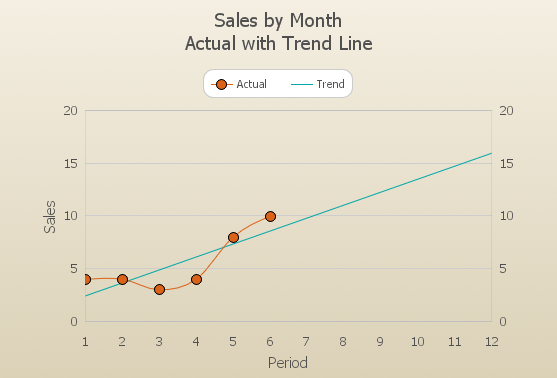Sometimes as software developers we get caught up in the details of collecting, storing, and displaying data. To our clients, this is rarely the ultimate goal. Our clients want to use data to make better decisions that will increase profits, utilize resources more efficiently, and improve lives.

This is part one of a series of articles exploring techniques for getting more out of your data. In this series, we’ll show you how to implement a variety of practical forecasting models in FileMaker Pro. A few of the topics we have planned include:

• Moving averages
• Weighted averages
• Exponential smoothing
• Trend projections
• Seasonal trend projections

While there are a variety of ways to implement these kinds of algorithms, we’re going to focus on using methods that are easy to understand and implement. We’ll be using FileMaker 12’s ExecuteSQL function extensively because it’s ideal for computing sums and averages from sets of data. Later in the series, we’ll explore ways to optimize performance when working with larger sets of data.

One goal of this series is to share HOW to implement these algorithms. However, a more important goal is to look at WHY and WHEN to use these techniques. To make the techniques easier to understand, we’re going to use the classic lemonade stand as a case study.

At Skeleton Key (and our sister company Brightsource IT), we’re an “open book” company, so forecasting is ingrained in our culture. We continuously share all of the details about how the business is performing with every employee and we actively involve employees in planning and decision making. We believe that educating everyone in how the business operates and giving our employees incentives to think and act like owners are keys to our success.

In this first article, we’ll look at a method for computing a trend line from a set of known values. In this case, our known values will be the monthly sales for the first six months of operating our lemonade stand. The trend line will help us visually see whether sales are growing, stable, or shrinking over time. It also provides a simplistic way to forecast future sales. Here’s the data we’ll be using:Plotting this data with a line chart looks like this:The purpose of a trend line is to plot a straight line that goes through the “center” of the known data points. Obviously a straight line can’t go through all of these points, so what we want to calculate is the straight line that misses all of the points by the least amount. A common way to calculate this is the “least squares method”. The least squares method finds the line that minimizes the total of the vertical distances between the line and each of the known data points. Let’s see what the trend line looks like and then we’ll discuss how it is calculated.A straight line can be expressed as the equation, Y = a + bX, where a is the Y-axis intercept and b is the slope. In our example, X is the month or period and Y is the predicted sales value.

In order to keep this article focused on practical application, we’ll skip over some of the details of how a and b can be calculated from the known data points, but suffice it to say there are statistical formulas that make this relatively straightforward. If you’d like to read more about this, I’d recommend checking out this Wikipedia article as a starting point.

Here are the pieces we need in order to calculate a and b:

n = the number of periods that have known sales values
sum_xy = the total of each period value times its sales value
sum_x = the total of the period values
sum_xx = the total of each of the period values squared
avg_y = the average of the sales values
avg_x = the average of the period values

Let’s calculate these manually for our sample data:

n = 6
sum_xy = (1*4) + (2*4) + (3*3) + (4*4) + (5*8) + (6*10) = 137
sum_x = 1 + 2 + 3 + 4 + 5 + 6 = 21
sum_xx = (1*1) + (2*2) + (3*3) + (4*4) + (5*5) + (6*6) = 91
avg_y = (4 + 4 + 3 + 4 + 8 + 10) / 6 = 5.5
avg_x = (1 + 2 + 3 + 4 + 5 + 6) / 6 = 3.5

Each of these can be calculated easily with simple SQL queries using the COUNT, SUM, and AVG aggregate functions. For example, the query to calculate sum_xy is

SELECT SUM(period*sales)
FROM sales_number
WHERE sales IS NOT NULL

Let’s finish calculating our example manually. The formulas for a and b are:

B = (sum_xy – (n * avg_x * avg_y)) / (sum_xx – (n * avg_x^2))
A = avg_y – (B * avg_x)

Plugging in values from above, we get:

B = (137 – (6 * 3.5 * 5.5)) / (91 – (6 * 3.5^2)) = 1.2286
A = 5.5 – (1.2286 * 3.5) = 1.2

Using the Y = a + bX equation we can then calculate the forecasted sales for July (period 7) as:

Y = 1.2 + (1.2286 * 7) = 9.8

To calculate all of this in FileMaker, we’ll use a Let function to calculate each of the components and then return the final value:

```Let( [

n = ExecuteSQL("SELECT COUNT(sales) FROM sales_number";"";"");

sum_xy = ExecuteSQL("SELECT SUM(period*sales) FROM sales_number WHERE sales IS NOT NULL";"";"");

sum_x = ExecuteSQL("SELECT SUM(period) FROM sales_number WHERE sales IS NOT NULL";"";"");

sum_y = ExecuteSQL("SELECT SUM(sales) FROM sales_number WHERE sales IS NOT NULL";"";"");

sum_xx = ExecuteSQL("SELECT SUM(period**2) FROM sales_number WHERE sales IS NOT NULL";"";"");

avg_y = ExecuteSQL("SELECT AVG(sales) FROM sales_number WHERE sales IS NOT NULL";"";"");

avg_x = ExecuteSQL("SELECT AVG(period) FROM sales_number WHERE sales IS NOT NULL";"";"");

B = (sum_xy - (n* avg_x * avg_y)) / (sum_xx - n * avg_x^2);
A = avg_y - (B * avg_x);
Y = A + (B * sales_number::period)

];
Y
)```

By extending our found set of records to include periods for the entire year, we can see how the trend line extends into the future.In this first article of our Lemonade Stand series, we’ve explored how to use simple linear regression to calculate a value at any point based on the trend established by a set of known values. This technique can be very useful for projecting values like future sales, inventory levels, or anything that is likely to follow a linear trend.

You might expect that sales for a lemonade stand could experience some seasonal variation. In a future article, we’ll explore how to adjust our forecast based on a seasonal index as well as how to calculate the seasonal index values based on historical data from previous years.

In Part 2, we’ll take a quick look at how to modify our trend line calculation to work with a period that is a date rather than a number.

We’re working on an example file that covers all of the techniques for this series. If you’d like to get a copy of the example file or if you’ve found this article useful and would like to see the series continue, please let us know at info@skeletonkey.com.

Greg Lane is VP of Application Development at Skeleton Key and a FileMaker Certified Developer.